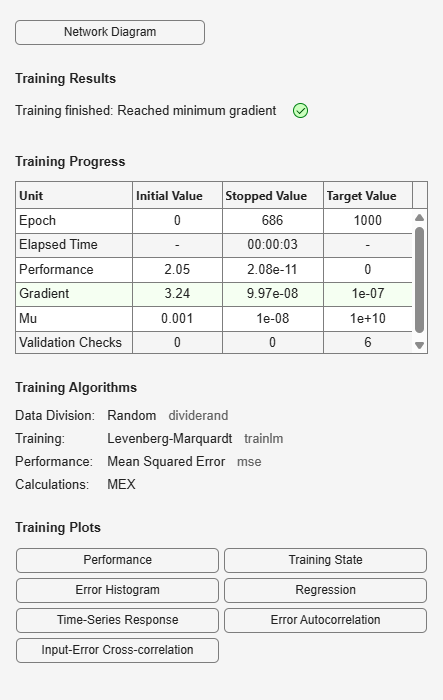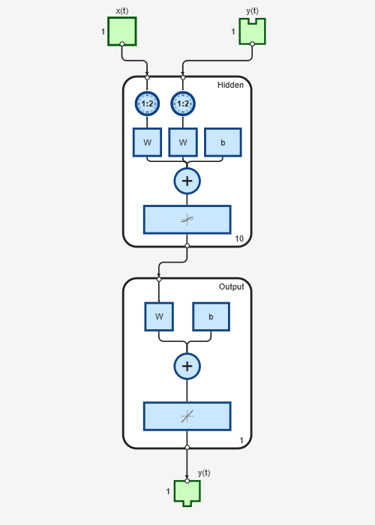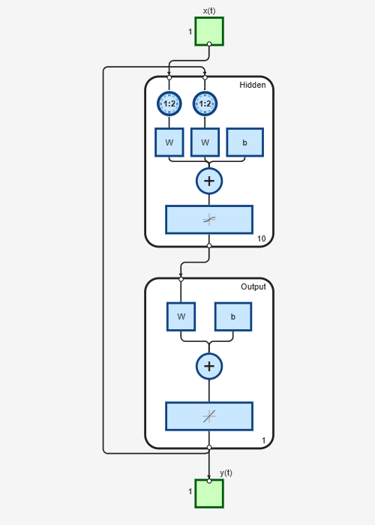# closeloop

Convert neural network open-loop feedback to closed loop

## Syntax

```net = closeloop(net) [net,xi,ai] = closeloop(net,xi,ai) ```

## Description

`net = closeloop(net)` takes a neural network and closes any open-loop feedback. For each feedback output `i` whose property `net.outputs{i}.feedbackMode` is `'open'`, it replaces its associated feedback input and their input weights with layer weight connections coming from the output. The `net.outputs{i}.feedbackMode` property is set to `'closed'`, and the `net.outputs{i}.feedbackInput` property is set to an empty matrix. Finally, the value of `net.outputs{i}.feedbackDelays` is added to the delays of the feedback layer weights (i.e., to the delays values of the replaced input weights).

`[net,xi,ai] = closeloop(net,xi,ai)` converts an open-loop network and its current input delay states `xi` and layer delay states `ai` to closed-loop form.

## Examples

### Convert NARX Network to Closed-Loop Form

This example shows how to design a NARX network in open-loop form, then convert it to closed-loop form.

```[X,T] = simplenarx_dataset; net = narxnet(1:2,1:2,10); [Xs,Xi,Ai,Ts] = preparets(net,X,{},T); net = train(net,Xs,Ts,Xi,Ai);````view(net)````Yopen = net(Xs,Xi,Ai); net = closeloop(net); view(net)``````[Xs,Xi,Ai,Ts] = preparets(net,X,{},T); Yclosed = net(Xs,Xi,Ai);```

### Convert Delay States

For examples on using `closeloop` and `openloop` to implement multistep prediction, see `narxnet` and `narnet`.

## Version History

Introduced in R2010b